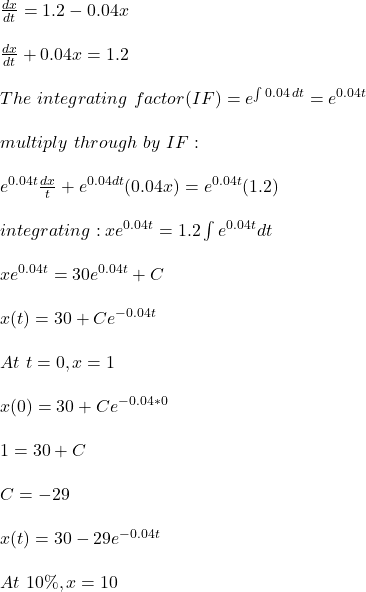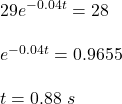## A nitric acid solution flows at a constant rate of 6 L/min into a large tank that initially held 200 L of a 0.5% nitric acid solution. The s

Question

A nitric acid solution flows at a constant rate of 6 L/min into a large tank that initially held 200 L of a 0.5% nitric acid solution. The solution inside the tank is kept well stirred and flows out of the tank at a rate of 8 L/min. If the solution entering the tank is 20% nitric acid, determine the volume of nitric acid in the tank after t min. When will the percentage of nitric acid in the tank reach 10%?

in progress 0
7 months 2021-07-17T09:48:57+00:00 1 Answers 0 views 0

## Answers ( )

0.88 s

Step-by-step explanation:

Let x(t) be the volume of nitric acid  measured in liters after t minute. Therefore the rate of change in the volume of nitric acid (dx / dt) is:

dx / dt = rate of input of nitric acid – rate of output of nitric acid

rate of input of nitric acid = 6 L/min * 20% nitric acid / 100% solution = 1.2 L/min

rate of output of nitric acid = 8 L/min * x L / 200 L = 0.04x L/minThe percentage of nitric acid in the tank reach 10% at 0.88 s